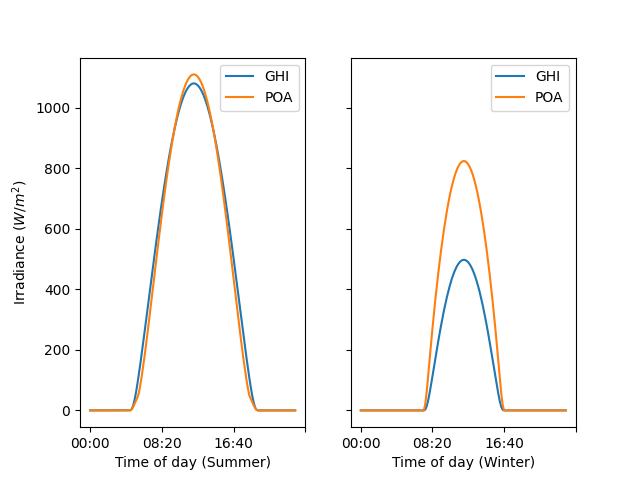# GHI to POA Transposition#

Example of generating clearsky GHI and POA irradiance.

This example shows how to use the `pvlib.location.Location.get_clearsky()` method to generate clearsky GHI data as well as how to use the `pvlib.irradiance.get_total_irradiance()` function to transpose GHI data to Plane of Array (POA) irradiance.

```from pvlib import location
import pandas as pd
from matplotlib import pyplot as plt

# For this example, we will be using Golden, Colorado
tz = 'MST'
lat, lon = 39.755, -105.221

# Create location object to store lat, lon, timezone
site = location.Location(lat, lon, tz=tz)

# Calculate clear-sky GHI and transpose to plane of array
# Define a function so that we can re-use the sequence of operations with
# different locations
# Creates one day's worth of 10 min intervals
times = pd.date_range(date, freq='10min', periods=6*24,
tz=site_location.tz)
# Generate clearsky data using the Ineichen model, which is the default
# The get_clearsky method returns a dataframe with values for GHI, DNI,
# and DHI
clearsky = site_location.get_clearsky(times)
# Get solar azimuth and zenith to pass to the transposition function
solar_position = site_location.get_solarposition(times=times)
# Use the get_total_irradiance function to transpose the GHI to POA
surface_tilt=tilt,
surface_azimuth=surface_azimuth,
dni=clearsky['dni'],
ghi=clearsky['ghi'],
dhi=clearsky['dhi'],
solar_zenith=solar_position['apparent_zenith'],
solar_azimuth=solar_position['azimuth'])
# Return DataFrame with only GHI and POA
return pd.DataFrame({'GHI': clearsky['ghi'],

# Get irradiance data for summer and winter solstice, assuming 25 degree tilt
# and a south facing array

# Convert Dataframe Indexes to Hour:Minute format to make plotting easier

# Plot GHI vs. POA for winter and summer
fig, (ax1, ax2) = plt.subplots(1, 2, sharey=True)
ax1.set_xlabel('Time of day (Summer)')
ax2.set_xlabel('Time of day (Winter)')